# Test for values in WordPress custom field shortcode

You can use a conditional shortcode to test a text or integer value returned by the shortcode for a WordPress custom field.

For example, you can test a shortcode with a statement such as "If the value of this shortcode is "coffee" then display this text, or else display this other text," or "If the value of this custom field shortcode is less than 2, then display this text or else display this other text."

The syntax is the same as for the Boolean-If and the if-else conditionals shortcode statements, with extra parameters:

[wpbb-if some-field-connection-shortcode
exp='<operator>' value='<value>']
Display this text when the value test is true.
[wpbb-else]Display this text when the value test is false.
[/wpbb-if]

The `exp` parameter is the operator, with the following values:

• For text strings, you can use the following operators:
• `equals`
• `notequals`
• For integers, you can use the following operators:
• `equals`
• `notequals`
• `greater`
• `greaterequals`
• `less`
• `lessequals`

The `value` parameter is the value that you want to test for. This can be a text string or an integer. Note that you can test for a date value as long as it is in the form YYYYMMDD, so the value can be compared as an integer.

##### Note

The `value` parameter is case-sensitive.

## Value expression examples#

The following examples are written so the actual value in the post being previewed is displayed for comparison. These examples are based on three WordPress custom fields with the following names:

• `wp_text_value`
• `wp_number_value`
• `wp_date`

Custom WordPress fields don't have data types, but these three fields were named for testing purposes to indicate what the value would be: a text string, an integer, or a date in the format YYYYMMDD.

This code was inserted into an HTML module in a Singular Themer layout to test values for WordPress custom fields that had been added to those posts. Look at the output in each example to help understand the code.

#### 1. Text `equals` example#

1 equals test<br>
[wpbb-if post:custom_field key='wp_text_value' exp='equals' value='Yay']
TRUE: Custom field value equals test value "Yay"<br>
wp_text_value is "[wpbb post:custom_field key='wp_text_value']"
[wpbb-else]FALSE: wp_text_value doesn't equal test value "Yay"<br>
wp_text_value is "[wpbb post:custom_field key='wp_text_value']"
[/wpbb-if]

Output: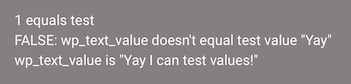#### 2. Text `notequals` test example#

2 text notequals test<br>
[wpbb-if post:custom_field key='wp_text_value' exp='notequals' value='terrible']
TRUE: wp_text_value doesn't equal test value "terrible"<br>
wp_text_value is "[wpbb post:custom_field key='wp_text_value']"
[/wpbb-if]

Output: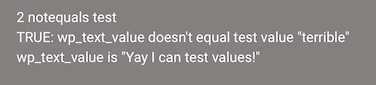#### 3. Number `equals` test example#

3 number equals test<br>
[wpbb-if post:custom_field key='wp_number_value' exp='equals' value='28883']
TRUE: wp_number_value equals test value "28883".<br>
The number field value is [wpbb post:custom_field key='wp_number_value']
[wpbb-else]FALSE: [wpbb post:custom_field key='wp_number_value'] doesn't equal test value "28883".
[/wpbb-if]

Output: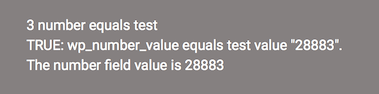#### 4. Number `greater` test example#

4 number greater test<br>
[wpbb-if post:custom_field key='wp_number_value' exp='greater' value='28883']
TRUE: wp_number_value is greater than test value "28883".<br>
wp_number_value is [wpbb post:custom_field key='wp_number_value']
[wpbb-else]FALSE: Custom field wp_number_value [wpbb post:custom_field key='wp_number_value'] is not greater than test value "28883".
[/wpbb-if]

Output: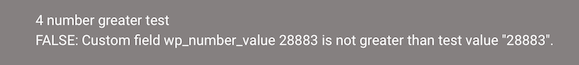#### 5. Number `greaterequals` test#

5 number greaterequals test<br>
[wpbb-if post:custom_field key='wp_number_value' exp='greaterequals' value='28883']
TRUE: wp_number_value is greater than or equals test value "28883".<br>
wp_number_value is [wpbb post:custom_field key='wp_number_value']
[wpbb-else]FALSE: Custom field wp_number_value [wpbb post:custom_field key='wp_number_value'] isn't greater than or equals test value "28883".
[/wpbb-if]

Output: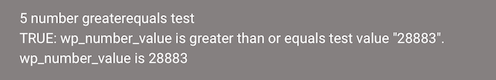#### 6. Date `equals` test#

6 date equals test<br>
[wpbb-if post:custom_field key='wp_date' exp='equals' value='201801']
TRUE: wp_date equals test value "201801".<br>
wp_date is [wpbb post:custom_field key='wp_date']
[wpbb-else]FALSE: wp_date doesn't equal test value "201801".<br>
wp_date is [wpbb post:custom_field key='wp_date']
[/wpbb-if]

Output: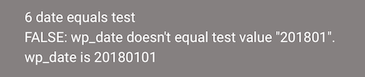#### 7. Date `less` test#

7 date less test<br>
[wpbb-if post:custom_field key='wp_date' exp='less' value='20171228']
TRUE: wp_date is less than test value "20171228".<br>
wp_date is [wpbb post:custom_field key='wp_date']
[wpbb-else]FALSE: wp_date isn't less than test value "20171228".<br>
wp_date is [wpbb post:custom_field key='wp_date']
[/wpbb-if]

Output: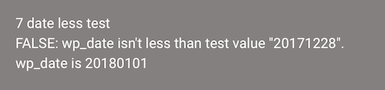#### 8. Date `lessequals` test#

8 date lessequals test<br>
[wpbb-if post:custom_field key='wp_date' exp='lessequals' value='20180101']
TRU>E: wp_date is less than or equals than test value "20180101".<br>
wp_date is [wpbb post:custom_field key='wp_date']
[wpbb-else]FALSE: [wpbb post:custom_field key='wp_date'] isn't less than or equals test value "20180101"
[/wpbb-if]

Output:Last updated on